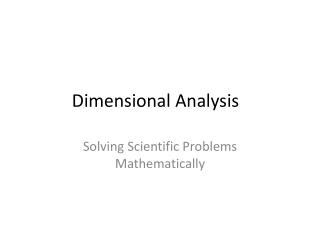# Dimensional Analysis - PowerPoint PPT PresentationDownload PresentationDimensional Analysis

Dimensional Analysis
Download Presentation## Dimensional Analysis

- - - - - - - - - - - - - - - - - - - - - - - - - - - E N D - - - - - - - - - - - - - - - - - - - - - - - - - - -
##### Presentation Transcript

1. Dimensional Analysis Solving Scientific Problems Mathematically

2. Dimensional Analysis is an orderly and systematic approach used to make MATHEMATICAL CONVERSIONS from one unit to another easy to accomplish. • Since measurements require both a NUMBER and a UNIT, this method is often referred to as the FACTOR LABEL method because UNITS MUST BE INCLUDED with each measurement.

3. Using the Factor Label Method • Let’s use a simple example to learn this method: • If someone were to tell you that they had 275 eggs and asked you how many dozen eggs that represented, how would you find that answer? • Before solving that problem, you would have to know that 12 eggs make up 1 DOZEN. • Once you knew that, solving the problem would be easy.

4. You would simple divide 275 by 12 to determine how many dozen eggs you have. • Written out mathematically, your calculation would look something like this: • 275eggs1 dozen=22.9 dozen • 12eggs • From this set up, do you see any UNITS that could be canceled DIAGONALLY?

5. 275 eggs 1 dozen = 22.9 dozen • 12 eggs • Notice the EGGS canceled diagonally, leaving the final unit of DOZENS as the only unit for the answer. • This is called the FACTOR LABEL METHOD: • Conversions involve NUMBERS with UNITS. • What was the relationship between 12 eggs and 1 dozen?

6. Since 12 eggs make 1 dozen, this relationship is called a CONVERSION FACTOR. • A conversions factor represents a number of smaller units that make 1 of a larger unit, as in this case 12 eggs (smaller units) = 1 dozen (larger unit). • Dimensional Analysis is written using a CROSS-BRACKET like this:

7. A dimensional analysis always begins with WHAT YOU ALREADY KNOW and is written in the upper corner of the frame: = X This is usually what’s GIVEN IN THE PROBLEM. • In our previous example, we were given 275 eggs.

8. Generally NOTHING (put an X in it) is written in the bottom of the first frame: 275 eggs • X • The SECOND FRAME contains the CONVERSION FACTOR: 12 EGGS = 1 DOZEN

9. How do you know which value GOES ON TOP and which value GOES ON BOTTOM? That’s easy….when everything is added to the frame, ALL UNITS EXCEPT THE FINAL ONE MUST CANCEL DIAGONALLY! • If they do not, something is in the wrong place!

10. EXAMPLE • Now let’s set up our egg problem using DIMENSIONAL ANALYSIS: 275 EGGS 1 DOZEN 275 Dozen 12 = 12 EGGS X Once all the numbers are in place and UNITS ARE CANCELED…everything ACROSS the TOP LINE is MULTIPLIED, then everything ACROSS the BOTTOM LINE is MULTIPLIED, then the TOP is DIVIDED by the BOTTOM for the FINAL ANSWER.

11. PRACTICE PROBLEM • There are 39.37 inches in 1 meter. Set up a DIMENSIONAL ANALYSIS problem to convert 8.75 meters to inches.

12. 8.75 meters 39.37 inches 318.601 inches 1 = 1 meter X Once all the numbers are in place and UNITS ARE CANCELED…everything ACROSS the TOP LINE is MULTIPLIED, then everything ACROSS the BOTTOM LINE is MULTIPLIED, then the TOP is DIVIDED by the BOTTOM for the FINAL ANSWER. = 318.601 INCHES

13. YOUR TURNSHOW YOUR WORK as DIMENSIONAL ANALYSIS • Problem #1 How many inches are there in 45 meters if each meter is 39.37 inches? • How many ounces are contained in 37.5 pounds assuming 1 pound is 16 ounces?

14. Practice with CONVERSION FACTORS • Below are some common CONVERSION FACTORS. If you do NOT know these, use Google or another search engine to find them. • 1. 1 foot = _____ inches 2. 1 meter = ______ centimeter • 3. 1 pound = ______ ounces 4. 1 pound = ______ grams • 5. 1 mile = ______ feet 6. 1 mile = _______ kilometers • 7. 1 liter = ______ ounces 8. 1 gallon = _______ ounces • 9. 1 ton = _______ pounds 10. 1 meter = ______ millimeters • 11. 1 gallon = ______ milliliters 12. 1 inch = _____ centimeters